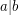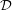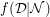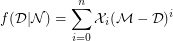# mimic function

## Distribution and upper bound of mimic numbers ★★

Author(s): Bhattacharyya

Problem

Let the notationdenote ''divides''. The mimic function in number theory is defined as follows .

Definition   For any positive integerdivisible by, the mimic function,, is given by,By using this definition of mimic function, the mimic number of any non-prime integer is defined as follows .

Definition   The numberis defined to be the mimic number of any positive integer, with respect to, for the minimum value of which.

Given these two definitions and a positive integer, find the distribution of mimic numbers of those numbers divisible by.

Again, find whether there is an upper bound of mimic numbers for a set of numbers divisible by any fixed positive integer.

Keywords: Divisibility; mimic function; mimic number